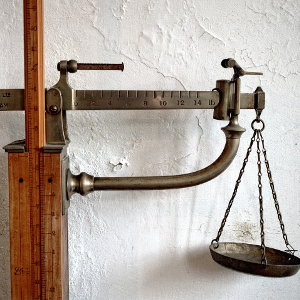# Kg to Lb and Oz Calculator / Kilograms to Pounds & Ounces Unit Converter

Share:

Kilograms
kg
Pounds
lb
Ounce
oz

Pounds
lb
Ounce
oz
Kilograms
kg

Result:

Kilograms
kg
Pounds
lb

Pounds
lb
Kilograms
kg

Result:

Kilograms
kg
Ounces
oz

Ounces
oz
Kilograms
kg

Result:

Pounds
lb
Ounces
oz

Ounces
oz
Pounds
lb

Result:

## Kilograms, Pounds & Ounces Conversion Chart

### Generating and Printing a Chart

1. Select a type of conversion you want from the drop down box Conversion Type.
2. Select the decimal place rounding from the Rounding drop down.
3. Enter the Minimum Weight you desire to start the conversion chart. The unit of weight will be either kilograms(kg), pounds(lb) or ounces(oz) based on the conversion type you have selected.
4. Enter the Interval between the weights. Example: 1, 10, 100, 1000, 0.5, 0.25, 0.001, etc...
5. Click the Generate Chart button to generate your desired chart. See list of charts you can generate.
6. Click the Print button to print the chart you have generated.

Conversion Type
Rounding
Start. Weight
Kg
Increment
Kg

Kilogram Pound & Ounce
1kg 2lb 3.274oz
2kg 4lb 6.548oz
3kg 6lb 9.822oz
4kg 8lb 13.096oz
5kg 11lb 0.37oz
6kg 13lb 3.644oz
7kg 15lb 6.918oz
8kg 17lb 10.192oz
9kg 19lb 13.466oz
10kg 22lb 0.74oz
11kg 24lb 4.014oz
12kg 26lb 7.288oz
13kg 28lb 10.562oz
14kg 30lb 13.835oz
15kg 33lb 1.109oz
16kg 35lb 4.383oz
17kg 37lb 7.657oz
18kg 39lb 10.931oz
19kg 41lb 14.205oz
20kg 44lb 1.479oz
21kg 46lb 4.753oz
22kg 48lb 8.027oz
23kg 50lb 11.301oz
24kg 52lb 14.575oz
25kg 55lb 1.849oz
Kilogram Pound & Ounce
26kg 57lb 5.123oz
27kg 59lb 8.397oz
28kg 61lb 11.671oz
29kg 63lb 14.945oz
30kg 66lb 2.219oz
31kg 68lb 5.493oz
32kg 70lb 8.767oz
33kg 72lb 12.041oz
34kg 74lb 15.315oz
35kg 77lb 2.589oz
36kg 79lb 5.863oz
37kg 81lb 9.137oz
38kg 83lb 12.411oz
39kg 85lb 15.685oz
40kg 88lb 2.958oz
41kg 90lb 6.232oz
42kg 92lb 9.506oz
43kg 94lb 12.78oz
44kg 97lb 0.054oz
45kg 99lb 3.328oz
46kg 101lb 6.602oz
47kg 103lb 9.876oz
48kg 105lb 13.15oz
49kg 108lb 0.424oz
50kg 110lb 3.698oz
Kilogram Pound & Ounce
51kg 112lb 6.972oz
52kg 114lb 10.246oz
53kg 116lb 13.52oz
54kg 119lb 0.794oz
55kg 121lb 4.068oz
56kg 123lb 7.342oz
57kg 125lb 10.616oz
58kg 127lb 13.89oz
59kg 130lb 1.164oz
60kg 132lb 4.438oz
61kg 134lb 7.712oz
62kg 136lb 10.986oz
63kg 138lb 14.26oz
64kg 141lb 1.534oz
65kg 143lb 4.808oz
66kg 145lb 8.081oz
67kg 147lb 11.355oz
68kg 149lb 14.629oz
69kg 152lb 1.903oz
70kg 154lb 5.177oz
71kg 156lb 8.451oz
72kg 158lb 11.725oz
73kg 160lb 14.999oz
74kg 163lb 2.273oz
75kg 165lb 5.547oz
Kilogram Pound & Ounce
76kg 167lb 8.821oz
77kg 169lb 12.095oz
78kg 171lb 15.369oz
79kg 174lb 2.643oz
80kg 176lb 5.917oz
81kg 178lb 9.191oz
82kg 180lb 12.465oz
83kg 182lb 15.739oz
84kg 185lb 3.013oz
85kg 187lb 6.287oz
86kg 189lb 9.561oz
87kg 191lb 12.835oz
88kg 194lb 0.109oz
89kg 196lb 3.383oz
90kg 198lb 6.657oz
91kg 200lb 9.931oz
92kg 202lb 13.204oz
93kg 205lb 0.478oz
94kg 207lb 3.752oz
95kg 209lb 7.026oz
96kg 211lb 10.3oz
97kg 213lb 13.574oz
98kg 216lb 0.848oz
99kg 218lb 4.122oz
100kg 220lb 7.396oz

## Types Of Kilograms, Pounds & Ounces Conversion Charts

Below are the types of kg, lbs and oz conversion charts or tables you can generate. You can choose these charts by changing the options in Conversion Type drop down field.

• Kilograms to Pounds & Ounces (kg to lb and oz) conversion chart.
• Kilograms to Pounds (kg to lb) conversion chart.
• Kilograms to Ounces (kg to oz) conversion chart.
• Pounds to Ounces (lb to oz) conversion chart.
• Pounds to Kilograms (lb to kg) conversion chart.
• Ounces to Kilograms (oz to kg) conversion chart.
• Ounces to Pounds (oz to lb) conversion chart.

## Kilogram

Kilogram is the basic unit of mass in metric system. Kilogram is also represented as kilo and abbreviated as (kg). One kilogram is approximately equivalent to one liter of pure water. Apart from kilogram, the other commonly used metric mass units are grams and milligram. 1 kilogram is 1000 grams and 1 gram is 1000 milligrams.

## Pound

Pound is the basic unit of mass in imperial system. Pound is also represented as avoirdupois pound and abbreviated as (lb) or (lbs). One pound is equal to 0.45359237 kilograms.

## Ounce

Ounce is another unit of mass in imperial system. Ounce is represented as avoirdupois ounce and abbreviated as (oz). 16 Ounces makes 1 pound.

## Kilograms, Pounds & Ounces Conversion• 1 Kilogram = 2.2046226218487758072297380135 Pounds.
• 1 Kilogram = 35.273961949580412915675808215 Ounces.
• 1 Kilogram = 2 Pounds and 3.273961949580412915675808216 Ounces.
• 1 Pound = 16 Ounces.
• 1 Pound = 0.45359237 Kilograms.
• 1 Ounce = 0.0625 Pounds.
• 1 Ounce = 0.028349523125 Kilograms.

### Formula for converting kilogram to pounds

1 kilogram is equal to 2.204622621849 pounds or lbs.

If M(kg) represents mass in kilograms and M(lb) represents mass in pounds, then the formula for converting kg to lbs is:

M(lb) = 2.204622621849 × M(kg)

#### Example

To convert 3 kilograms to pounds:
M(kg) = 3

M(lb) = 2.204622621849 × M(kg) = 2.204622621849 × 3 = 6.613867865546 lbs

### Formula for converting kilogram to ounces

1 kilogram is equal to 2.204622621849 pounds or lbs and 1 pound is equal to 16 ounces or oz.

If M(kg) represents mass in kilograms, M(lb) represents nass in pounds and M(oz) represents mass in ounces, then the formula for converting kg to oz is:

M(oz) = 16 × M(lb)

M(lb) = 2.204622621849 × M(kg)

So, M(oz) = 16 × 2.204622621849 × M(kg) = 35.27396194958 × M(kg)

M(oz) = 35.27396194958 × M(kg)

#### Example

To convert 3 kilograms to ounces:
M(kg) = 3

M(oz) = 35.27396194958 × M(kg) = 35.27396194958 × 3 = 105.821885848741 oz